Analysis WebNotes
arrow_back arrow_forward

# Class Contents

## Compactness and Sequential Compactness are Equivalent (continued)

### On with the proof of Theorem 6.24

Our standing assumption throughout the remainder of this section will be that X is a metric space and that K is a sequentially compact subset of X. Our aim will be to show that K is compact. To this end, suppose further that we have been presented with a fixed open cover U of K. Our job will be to show that we can pick out a finite cover of K consisting of points of U.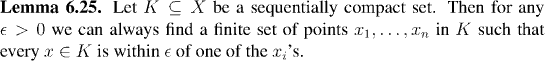Claim: We can use Lemma 6.25 to find a sequence (xn) of points in K which can get arbitrarily close to any point in K. To do this, we use the following construction: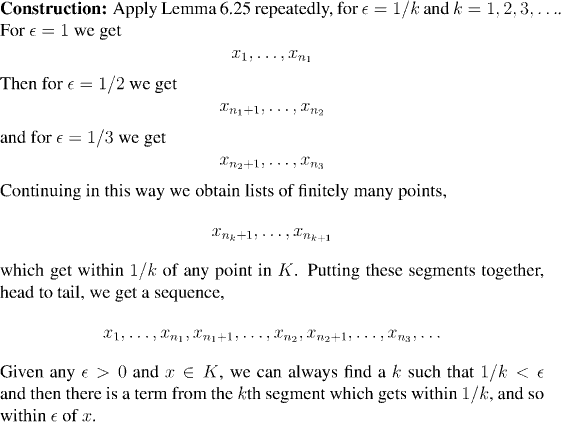Remark:

We call the set of points (xn) a countable, dense set.Now we use the xn to select out a collection of open sets from the open cover which we can work with.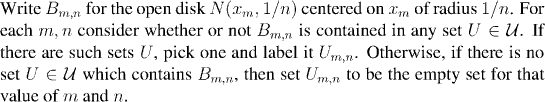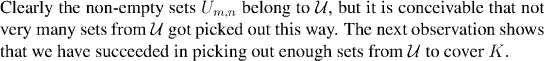Claim: The sets U{m,n} cover K.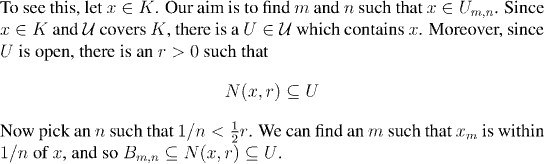The picture shows the details of this clearly: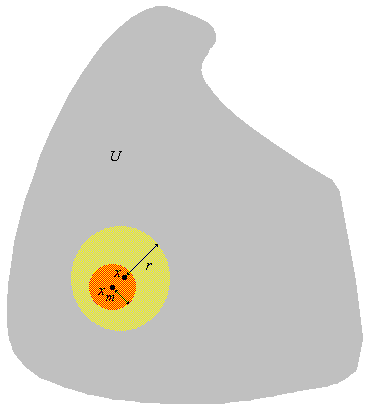Question: Work out the details of why B{m,n} is contained in U.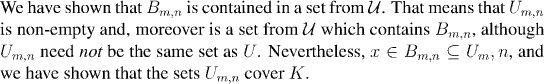We are now ready for the main part of the argument. We shall show that finitely many of the U{m,n} will cover K. Since the non-empty U{m,n} all come from U, this will prove our result.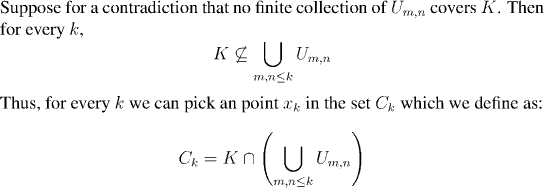Factoid: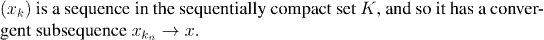Factoid: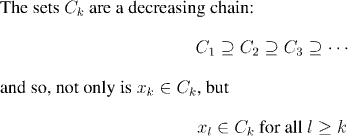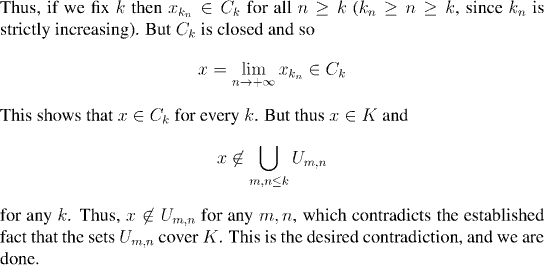The next class, which is the last one of this chapter, will introduce a new property of metric spaces: completeness. This allows us to answer the question "When does a sequence converge?", without knowing in advance what it is supposed to converge to.